# Deducing the Baker-Campbell-Hausdorff formula from associative algebra manipulation

(diff) ← Older revision | Latest revision (diff) | Newer revision → (diff)

## Statement

### Infinite version

The Baker-Campbell-Hausdorff formula in the simply connected case (and hence also the nilpotent case, at least in large characteristic) can be computed by the following procedure.

• Start with the power series for the exponential function$\exp(X)$ and$\exp(Y)$ viewed as elements of the formal power series ring$\mathbb{Q}\langle X,Y \rangle$ with$X,Y$ non-commuting variables:$\! \exp(X) = \sum_{k=0}^\infty \frac{X^k}{k!} = 1 + X + \frac{X^2}{2!} + \frac{X^3}{3!} + \dots$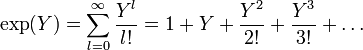$\! \exp(Y) = \sum_{l=0}^\infty \frac{Y^l}{l!} = 1 + Y + \frac{Y^2}{2!} + \frac{Y^3}{3!} + \dots$

Now, multiply both of these:$\! \exp(X)\exp(Y) = \sum_{k=0}^\infty \sum_{l=0}^\infty \frac{X^kY^l}{k!l!}$

Subtract 1 and define$W = \exp(X)\exp(Y) - 1 = (\exp(X) - 1)(\exp(Y) - 1) + (\exp(X) - 1) + (\exp(Y) - 1)$. We have:$\! W = \sum_{k,l \ge 0, 0 < k + l} \frac{X^kY^l}{k!l!} = \sum_{n=1}^\infty \frac{1}{n!} \sum_{k=0}^n \binom{n}{k}X^kY^{n-k}$

The Baker-Campbell-Hausdorff formula is obtained by applying the power series for$\log(1 + W)$ and plugging the above expression for$W$ into that. Ultimately, we have to convert terms that are obtained in terms of$X,Y$ into commutators and iterated commutators.

### Truncated version for nilpotent groups

In the case of a group of nilpotency class at most$c$, we can simplify the computations above as follows: we can work within a ring where all products of length$c + 1$ or more are zero. Thus, in here, we can assume that:$\! \exp(X) = \sum_{k=0}^{c-1} \frac{X^k}{k!} = 1 + X + \frac{X^2}{2!} + \frac{X^3}{3!} + \dots + \frac{X^{c-1}}{(c - 1)!}$$\! \exp(Y) = \sum_{l=0}^{c-1} \frac{Y^l}{l!} = 1 + Y + \frac{Y^2}{2!} + \frac{Y^3}{3!} + \dots + \frac{Y^{c-1}}{(c - 1)!}$

Now, multiply both of these and remove all terms where the total exponent is$c + 1$ or more:$\! \exp(X)\exp(Y) = \sum_{k,l \ge 0, k + l \le c} \frac{X^kY^l}{k!l!}$

Subtract 1 and define$W = \exp(X)\exp(Y) - 1$. We have:$\! W = \sum_{k,l \ge 0, 0 < k + l \le c} \frac{X^kY^l}{k!l!} = \sum_{n=1}^c \frac{1}{n!} \sum_{k=0}^n \binom{n}{k} X^kY^{n-k}$

Now apply the power series for$\log(1 + W)$. Note that this power series again can be terminated after$c$ terms.

## Particular cases

We consider the cases for various nilpotent groups for fixed bounds on nilpotency class.

### Case of abelian group

The short version is as follows. Using the quick formula for$W$, we obtain:$W = X + Y$

Since we are in the abelian case,$W^2 = 0$, so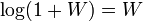$\log(1 + W) = W$, so we get that the Baker-Campbell-Hausdorff formula gives:$\! X + Y$

### Case of class two

#### Quick version

By the quick formula, we have: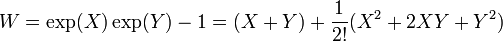$W = \exp(X)\exp(Y) -1 = (X + Y) + \frac{1}{2!}(X^2 + 2XY + Y^2)$

Further, since we are in class two,$W^3 = 0$, so we get:$\log(1 + W) = W - \frac{W^2}{2} = (X + Y) + \frac{1}{2!}(X^2 + 2XY + Y^2) - \frac{1}{2}((X + Y) + \frac{1}{2!}(X^2 + 2XY + Y^2))^2$

In the second square term, the class two condition means that the only surviving part is$(X + Y)^2$, so we get:$\log(1 + W) = (X + Y) + \frac{1}{2!}(X^2 + 2XY + Y^2) - \frac{1}{2}(X + Y)^2$

Simplify, and get:$(X + Y) + \frac{1}{2}(X^2 + 2XY + Y^2 - X^2 - XY - YX - Y^2)$

This further simplifies to:$(X + Y) + \frac{1}{2}(XY - YX)$

This can be rewritten as:$(X + Y) + \frac{1}{2}[X,Y]$

#### Detailed version

In this case, we work with non-commuting variables$X,Y$ such that$X^3 = X^2Y = XYX = XY^2 = YX^2 = YXY = Y^2X = Y^3 = 0$. Thus:$\! \exp(X) = 1 + X + \frac{X^2}{2}$$\! \exp(Y) = 1 + Y + \frac{Y^2}{2}$

We thus get:$\exp(X)\exp(Y) = \left(1 + X + \frac{X^2}{2}\right)\left(1 + Y + \frac{Y^2}{2}\right) = 1 + X + \frac{X^2}{2} + Y + XY + \frac{X^2Y}{2} + \frac{Y^2}{2} + \frac{XY^2}{2} + \frac{X^2Y^2}{4}$

We drop all products of degree three or more and rearrange the remaining terms to get:$\! \exp(X)\exp(Y) = 1 + X + Y + \frac{X^2}{2} + XY + \frac{Y^2}{2}$

We thus get:$\! W = \exp(X)\exp(Y) - 1 = X + Y + \frac{X^2}{2} + XY + \frac{Y^2}{2}$

Finally, we compute$\log(1 + W)$. We have:$\log(1 + W) = W - \frac{W^2}{2}$

We note that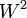$W^2$ is the same as the square of its linear part because the square of the degree two part, as well as products of the degree two and the linear part, are degree three or more and hence zero. Thus:$\log(1 + W) = X + Y + \frac{X^2}{2} + XY + \frac{Y^2}{2} - (X + Y)^2/2$

Simplifying, we get:$\log(1 + W) = X + Y + \frac{X^2 + 2XY + Y^2 - (X + Y)^2}{2}$

Now note that: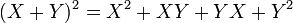$\! (X + Y)^2 = X^2 + XY + YX + Y^2$

Plugging this in, we get:$\log(1 + W) = X + Y + \frac{X^2 + 2XY + Y^2 - (X^2 + XY + YX + Y^2)}{2}$

Simplifying, we get:$\log(1 + W) = X + Y + \frac{XY - YX}{2}$

Rewrite$XY - YX = [X,Y]$ and we get the formula:$\! X + Y + \frac{1}{2}[X,Y]$

### Case of class three

By the quick formula, we have:$W = \exp(X)\exp(Y) -1 = (X + Y) + \frac{1}{2!}(X^2 + 2XY + Y^2) + \frac{1}{3!}(X^3 + 3X^2Y + 3XY^2 + Y^3)$

Since the class is three, we have$W^4 = 0$, hence we get:$\log(1 + W) = W - \frac{W^2}{2} + \frac{W^3}{3} = (X + Y) + \frac{1}{2!}(X^2 + 2XY + Y^2) + \frac{1}{3!}(X^3 + 3X^2Y + 3XY^2 + Y^3) - \frac{1}{2}((X + Y) + \frac{1}{2!}(X^2 + 2XY + Y^2) + \frac{1}{3!}(X^3 + 3X^2Y + 3XY^2 + Y^3))^2 + \frac{1}{3}((X + Y) + \frac{1}{2!}(X^2 + 2XY + Y^2) + \frac{1}{3!}(X^3 + 3X^2Y + 3XY^2 + Y^3))^3$

The calculations for the degree one and degree two parts proceed exactly as they did in the class two case (see Baker-Campbell-Hausdorff formula for nilpotency class two). We thus concentrate on the degree three part:$\mbox{Degree three part} = \frac{1}{6}(X^3 + 3X^2Y + 3XY^2 + Y^3) - \frac{1}{4}(X + Y)(X^2 + 2XY + Y^2) - \frac{1}{4}(X^2 + 2XY + Y^2) + \frac{1}{3}(X + Y)^3$$X^2 + 2XY + Y^2 = (X + Y)^2 + [X,Y]$

We similarly rewrite:$X^3 + 3X^2Y + 3XY^2 + Y^3 = (X + Y)^3 + 2X[X,Y] + [X,Y]X + 2[X,Y]Y + Y[X,Y]$

This can be further rewritten as:$X^3 + 3X^2Y + 3XY^2 + Y^3 = (X + Y)^3 + 3X[X,Y] + 3[X,Y]Y - [X,[X,Y]] + [Y,[X,Y]]$

Now, we plug these into the expression$\mbox{Degree three part} = \frac{1}{6}((X + Y)^3 + 3X[X,Y] + 3[X,Y]Y - [X,[X,Y]] + [Y,[X,Y]]) - \frac{1}{4}(X + Y)((X + Y)^2 + [X,Y]) - \frac{1}{4}((X + Y)^2 + [X,Y])(X + Y) + \frac{1}{3}(X + Y)^3$

We rearrange to obtain:$\mbox{Degree three part} = \left(\frac{1}{6} - \frac{1}{4} - \frac{1}{4} + \frac{1}{3}\right)(X + Y)^3 + \frac{1}{2}(X[X,Y] + [X,Y]Y) - \frac{1}{6}([X,[X,Y]] - [Y,[X,Y]]) - \frac{1}{4}(X + Y)[X,Y] - \frac{1}{4}([X,Y](X + Y))$

The$(X + Y)^3$ term has zero coefficient and disappears, and we are left with:$\mbox{Degree three part} = \frac{1}{2}(X[X,Y] + [X,Y]Y) - \frac{1}{6}([X,[X,Y]] - [Y,[X,Y]]) - \frac{1}{4}X[X,Y] - \frac{1}{4}Y[X,Y] - \frac{1}{4}[X,Y]X - \frac{1}{4}[X,Y]Y$

We now use that$Y[X,Y] = [X,Y]Y + [Y,[X,Y]]$ and$[X,Y]X = X[X,Y] - [X,[X,Y]]$ to get:$\mbox{Degree three part} = \frac{1}{2}(X[X,Y] + [X,Y]Y) - \frac{1}{6}([X,[X,Y]] - [Y,[X,Y]]) - \frac{1}{4}X[X,Y] - \frac{1}{4}[X,Y]Y - \frac{1}{4}[Y,[X,Y]] - \frac{1}{4}X[X,Y] + \frac{1}{4}[X,[X,Y]] - \frac{1}{4}[X,Y]Y$

Combining coefficients, we find that the coefficients on$X[X,Y]$ and$[X,Y]Y$ are zero, and we are left with:$\mbox{Degree three part} = - \frac{1}{6}([X,[X,Y]] - [Y,[X,Y]]) + \frac{1}{4}([X,[X,Y]] - [Y,[X,Y]])$

We simplify$1/4 - 1/6 = 1/12$ to get:$\mbox{Degree three part} = \frac{1}{12}([X,[X,Y]] - [Y,[X,Y]])$

Plug this back in to the formula, and get the overall formula:$X + Y + \frac{1}{2}[X,Y] + \frac{1}{12}([X,[X,Y]] - [Y,[X,Y]])$